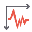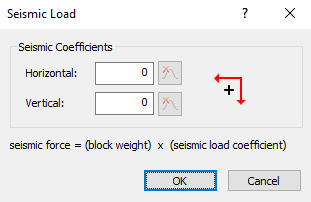Expert Modelling: Hands-on help from Rocscience Engineers. Register Here

## Search Results

The Seismic Loadoption in the Loading menu allows you to apply pseudo-static earthquake forces in the RocTopple limit equilibrium analysis by defining seismic coefficients for the Horizontal and / or Vertical directions. The Seismic Coefficients are dimensionless coefficients which represent the (maximum) earthquake acceleration as a fraction of the acceleration due to gravity. Typical values are in the range of 0.1 to 0.3.

1. Select Seismic Loadon the toolbar or the Loading menu.2. Enter the Horizontal and / or Vertical Seismic Coefficients.
Note the positive convention (horizontal positive is directed OUT of the slope and vertical positive is directed DOWN). You can enter negative coefficients if you want to apply the seismic force in the opposite direction (horizontal IN to the slope or vertical UP).
3. Select OK to include the pseudo-static seismic load in your analysis.

### SEISMIC COEFFICIENTS

When you apply the Seismic Coefficients, a pseudo-static force is applied through the centroid of each block in the horizontal and/or vertical directions. The applied force on each block is equal to:

Seismic Force = Seismic Coefficient * Block Weight * acceleration due to gravity

Note that the acceleration due to gravity (g = 9.81 m/s2 for metric units) is automatically accounted for in the analysis and does not need to be entered by the user. The Seismic Coefficients are dimensionless.

If you are carrying out a Probabilistic analysis, the Seismic Coefficients (horizontal or vertical) can be defined as random variables. See Seismic Load Statistics in a Probabilistic Analysis for more information.

### SENSITIVITY ANALYSIS

The Sensitivity analysis feature of RocTopple is useful to determine the effect of the Seismic Coefficients over any desired range of values. For example, you could enter a range of -0.5 to 0.5 to obtain a plot of Factor of Safety versus Seismic Coefficient over a range of possible values in both the positive and negative directions.